### Common Base ConversionsSource: https://www.tes.com/teaching-resource/0-9-digit-cards-6044974
When we represent numbers in mathematics, we utilize ten digits zero to nine to represent all numbers. In computer science, this is called the base 10 system because it has ten digits. There are three other common bases that are studied in C.S.: base 2, 8 and 16, with two, eight and sixteen digits, respectively. Before we examine each of these in more detail, your are probably asking...

Why do we need to learn manual base conversions?

Although humans read in base-10, computers read in base-2 as the internal mechanisms can only reliably provide two options (i.e. are switches) that make data storage only possible in binary. But as the number gets larger the characters of binary get much larger and unwieldy to work with when examining computer codes. In the past, base-8 was used to shorten these long strings of numbers into more manageable chunks, but today this base-16 has supplanted octal in this role because it is more efficient. Now, we will move onto how to convert between these systems. We will use "binary" as a base case from which you can access the other bases (i.e. you would not convert directly from decimal to octal, but from decimal to binary and then from binary to octal).

Binary and Decimal:

First we examine the question of how to convert between our native number system and binary.Source: https://www.geeksforgeeks.org/program-binary-decimal-conversion/
It is pretty intuitive to convert from binary to decimal. This is a base two system, and each extra digit represents a larger power of two. Since we only have two options, you will add either that power of two or not depending on whether the digit is zero or one.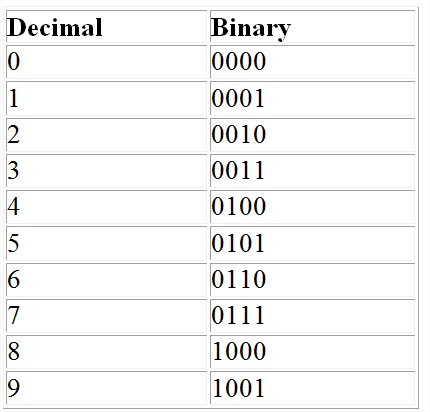Source: https://brilliant.org/wiki/binary-coded-decimal-or-bcd/
This table tells you how the decimal digits themselves are converted to binary numbers and vice-versa.Source: https://owlcation.com/stem/How-to-Convert-Decimal-to-Binary-and-Binary-to-Decimal
There is also a rather intuitive way to convert decimals to binary using long division as shown above. This also relies on the powers of two, but instead of multiplication as in the prior conversion it uses division.

Binary and Octal:Source: https://www.wikihow.com/Convert-Binary-to-Octal-Number
The relationship between octal and binary is based on this 4-2-1 relationship based on the cluster of the binary number, starting from the right in groups of three. If the final group (the most left-most or at the front of the number), does not have three, then you add on zeroes until you reach three. Then you write 4-2-1 and multiply by the available digits to generate the octal digits.Another example of binary to octal: https://circuitglobe.com/octal-to-binary-and-binary-to-octal-conversion-methods.htmlChart for the octal digits: https://www.youtube.com/watch?v=a4i1OnDtufc
Converting from octal to binary requires an intuitive sense of the top chart (or the 4-2-1 rule). You convert each octal digit back into its component three binary digits to get your final value.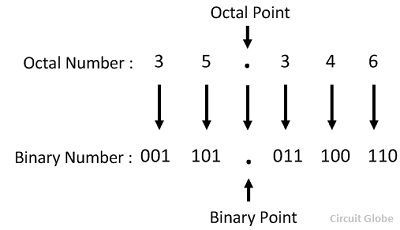Octal to Binary: https://circuitglobe.com/octal-to-binary-and-binary-to-octal-conversion-methods.html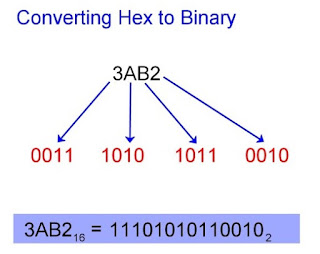Source: https://owlcation.com/stem/How-to-Convert-Hex-to-Binary-and-Binary-to-Hexadecimal
Hexadecimal relies on the very same principle as octal except with groups of four binary digits instead of three (thereby creating an 8-4-2-1 rule). The system adds extra digits A (10), B (11), C (12), D (13), E (14), F (15). I've added the decimal equivalents because it makes it easier to understand what they represent. This four-digit system allows for manual conversion forwards and backwards as with the previous system.

Extra Sources

### Text Symbols

This is an extended version of another user's text symbol sheet.

Ex. Symbol (to test copying/pasting): 囧

Lines:
┌ ┍ ┎ ┏ ┐ ┑ ┒ ┓ └ ┕
┖ ┗ ┘ ┙ ┚ ┛ ├ ┝ ┞ ┟
┠ ┡ ┢ ┣ ┤ ┥ ┦ ┧ ┨ ┩
┪ ┫ ┬ ┭ ┮ ┯ ┰ ┱ ┲ ┳
┴ ┵ ┶ ┷ ┸ ┹ ┺ ┻ ┼ ┽
┾ ┿ ╀ ╁ ╂ ╃ ╄ ╅ ╆ ╇
╈ ╉ ╊ ╋ ╌ ╍ ╎ ╏ ═ ║
╒ ╓ ╔ ╕ ╖ ╗ ╘ ╙ ╚ ╛
╜ ╝ ╞ ╟ ╠ ╡ ╢ ╣ ╤ ╥
╦ ╧ ╨ ╩ ╪ ╫ ╬

Arrows:
⏎ ➟ ➡ ➢ ➣ ➤ ➥ ➦ ➧ ➨
➚ ➘ ➙ ➛ ➜ ➝ ➞ ➸ ♐ ➲
➴ ➵ ➶ ➷ ➸➹ ➺ ➻ ➼ ➽
← ↑ → ↓ ↔ ↕ ↖ ↗↘ ↙
↚ ↛ ↜ ↝ ↞ ↟ ↠ ↡ ↢ ↣ ↤
↥ ↦ ↧ ↨ ➫ ➬ ➩ ➪ ➭ ➮ ➯ ➱
↩↪ ↫ ↬ ↭ ↮ ↯ ↰ ↱ ↲ ↳ ↴ ↵
↶↷ ↸ ↹ ↺ ↻ ↼ ↽ ↾ ↿ ⇀ ⇁ ⇂ ⇃
⇄ ⇅ ⇆ ⇇ ⇈ ⇉ ⇊ ⇋ ⇌ ⇍ ⇎ ⇏
⇐⇑ ⇒ ⇓ ⇔ ⇕ ⇖ ⇗ ⇘ ⇙ ⇚ ⇛
⇜ ⇝⇞ ⇟ ⇠ ⇡ ⇢ ⇣ ⇤ ⇥ ⇦ ⇧ ⇨ ⇩ ⇪

Zodiac:
♈ ♉ ♊ ♋
♌ ♍ ♎ ♏
♐ ♑ ♒ ♓

Shapes:
◉ ○ ◌ ◍ ◎ ● ◐
◑ ◒ ◓ ◔ ◕ ◖ ◗
❂ ☢ ⊗ ⊙ ◘ ◙ ◍

Chess:
♔ ♕ ♖ ♗ ♘ ♙
♚ ♛ ♜ ♝ ♞ ♟

Cards:
♤ ♧ ♡ ♢
♠ ♣ ♥ ♦

Bubble Numbers and Letters
Ⓐ Ⓑ Ⓒ Ⓓ Ⓔ Ⓕ Ⓖ Ⓗ Ⓘ Ⓙ Ⓚ Ⓛ Ⓜ Ⓝ Ⓞ Ⓟ Ⓠ Ⓡ Ⓢ Ⓣ Ⓤ Ⓥ Ⓦ Ⓧ Ⓨ Ⓩ

ⓐ ⓑ ⓒ ⓓ ⓔ ⓕ ⓖ ⓗ ⓘ ⓙ ⓚ ⓛ ⓜ ⓝ ⓞ ⓟ ⓠ ⓡ ⓢ ⓣ ⓤ ⓥ ⓦ ⓧ ⓨ ⓩ

➀ ➁ ➂ ➃ ➄ ➅ ➆ ➇ ➈ ➉

➊ ➋ ➌ ➍ ➎ ➏ ➐ ➑ ➒ ➓

Fingers:
☜ ☞ ☝
☚ ☛ ☟
✍ ✌

Weather:
☼ ☀ ☁ ☂ ☔
☄ ☾ ☽ ❄ ☃
☈ ⊙ ☉ ℃ ℉
° ❅ ✺ ϟ ☇

Faces:
☹ ☺ ☻ ت
ヅ ツ ッ シ
Ü ϡ ﭢ

Stars:
⋆ ✢ ✣ ✤ ✥ ❋
✦ ✧ ✩ ✰ ✪ ✫
✬ ✭ ✮ ✯ ❂ ✡
★ ✱ ✲ ✳ ✴
✵ ✶ ✷ ✸ ✹
✺ ✻ ✼ ❄ ❅
❆ ❇ ❈ ❉ ❊

Letters

A: Ⱥ Д ∆ ₳Ꭿ Ꭺ
B: ℬ Ᏸ 乃 ط ъ
C: 匚 ང Ꮳ ℂ
D: ƌ Ꭰ Ꮄ ⅅ ⅆ
E: ε ཇ Σ Ξ Ꭼ Ꮛ ⅇ
F: ན ƒ
G: Ꮹ ɠ ʛ Ꮆ Ᏻ
H: ħ ℌ Ꮋ Ꮒ Ᏺ ℍ
I: ༏ ༑ ༐ ⅈ Ꮠ
J: \uD840\uDCCC Ꭻ Ꮰ ℑ ⅉ
K: ƙ
L: ℒ Ꮮ ɭ Ꮭ ℓ ﷼
M: ཀ Ꮇ ᙢ ℳ
N: ₪ ת מ ה ས π ℵ
O: Ꮎ
P: ρ や 尸 Ꮅ
Q: Ꭴ
R: ર ℜ ཞ ༉ Ꭱ
S: Ꭶ
T: τ Ꮖ ϯ ד Ꮏ ե Ꭲ
U: Ꮜ
V: Ꭷ Ꮴ
W: Ꮤ ᙡ Ꮚ ש ཡ ш щ ϣ Ꮗ
X: ℵ א ж
Y: ע ч ɤ ɣ Ꮍ
Z: Հ ʑ Ꮓ ℤ

Extended Letters
A a à á â ä æ ã å ā Ⓐ ⓐ ɐ ɑ ɒ ∀ ∆ ∢ Գ Ճ ձ ਚ Д Ѧ ѧ Ѩ ѩ Ӑ ӑ Ӓ ӓ ӕ მ Λ ά α Ά ઊ ה ם À Á Â Ã Å Ā Ą ą Æ இ ค จ ถ อ ๙ ཐ བ Ꮜ Ꮑ Ꭺ Ꭿ ◬ ▵ ⋒ ⍶ ⍲ ⍱ ⍝ ⍙ ⍫ ⍺

ʙ ʚ ɮ ɞ ɓ ც Β βϐ ß Ɓ ƀ Ƅ ƅ ƃ Ƃ ฿ ๖ ธ ઉ Ь в ъ ь Ѣ ѣ Ҍ ҍ ճ B b ъႦႪ ს წ ც ხ ჱ ઇ Ḇ Ḃ Ḅ ḃ ḇ ḅ ß Ɓ ƃ Ƃ ℬ Ᏼ Ᏸ Ⓑ ⓑ

Ⓒ ⓒ C c Ç Ć ℂ ℃ ɔ ʗ ∁ ⊏ ⋐ ₠ ₡ ₢ ¢￠с Ҫ ҫ ე Ϛ ϲ Ç ç ć Ĉ ĉ Ċ ċ Č č Ḉ ḉ Ƈ Ɔ ƈ ༊ ང ༨ ℂ Ꮸ Ꮯ € ℭ ⊂

Ⓓ ⓓ ɖ ɗ ʠ ʣ ʤ ʥ ᵈ ժ ծ ձ ճ ԁ Ԁ მ ძ δ ઠ વ Ď ď Đ Ḋ Ḍ Ḏ Ḑ ḋ ḏ ḍ ḑ ḓ Ḓ Ð ð Ɖ Ɗ ƌ ƿ Ǆ ǅ ǆ Ǳ ǲ ǳ ฎ ๔ ⅆ ⅅ Ꮷ Ꭰ

Ⓔ ⓔ ℇ ℈ ℮ ℯ ℰ ɘ ə ɚ ɛ ɜ ɝ ʓ ʒᵉ ∃ ∄ ∈ ∉ ∊ ∌ ∋ ∍ ∑ ≡ ≆ ≋ ≇ ۼ ੬ ੩ Ѐ Ё Е Ш ѐ ё є Ҙ ҙ Ҽ ҽ Ҿ ҿ Ӗ Ӛ ӗ Ә ӛ Ӡ ӡ Ӭ ӭ Ԑ ԑ ६ ჳ ჴ Ε Ξ Σ έ ε ξ Έ દ ૩ c ૬ ૯ È è É é Ê ê Ë ë Ē ē Ĕ ĕ Ė ė Ę ę Ě ě Ȩ ȩ Ȅ ȅ Ȇ ȇ Ḕ ḕ Ḗ ḗ Ḙ ḙ Ḛ ḛ Ḝ ḝ Ẹ ẹ Ẻ ẻ Ẽ ẽ Ế ế Ề ề Ể ể Ễ ễ Ệ ệ Ǝ Ƹ ƹ Ǯ ǯ ༣ ཇ ཛ ཟ ℰ ⅇ ⅀ € Ꮛ Ꭼ

Ⓕ ⓕ ℉ ℱ ɟ ʄ ʆ∮ ∲ ∯ ∳ ∱ ⊧ ⊫₣ ƒ Բ բ Ғ ғ Ϝ Ḟ ḟ ẛ Ƒ ƒ ƭ F

Funky Letters
ჲ ჳ ხ Ꭰ Ꭱ Ꭲ Ꭳ Ꭴ Ꭵ Ꭶ Ꭷ Ꭿ Ꭾ Ꭽ Ꭼ Ꭻ Ꭺ Ꭹ Ꭸ Ꮀ Ꮁ Ꮂ Ꮃ Ꮄ Ꮅ Ꮆ Ꮇ Ꮈ Ꮉ Ꮊ Ꮋ Ꮌ Ꮍ Ꮎ Ꮏ Ꮐ Ꮑ Ꮒ Ꮓ Ꮔ Ꮖ Ꮕ Ꮗ Ꮘ Ꮙ Ꮚ Ꮛ Ꮜ Ꮝ Ꮞ Ꮟ Ꮠ Ꮡ Ꮢ Ꮣ Ꮤ Ꮥ Ꮦ Ꮧ Ꮨ Ꮩ Ꮪ Ꮫ Ꮬ Ꮭ Ꮮ Ꮯ Ꮰ Ꮱ Ꮲ Ꮳ Ꮴ Ꮵ Ꮶ Ꮷ Ꮿ Ꮾ Ꮽ Ꮼ Ꮻ Ꮺ Ꮸ Ᏸ Ᏹ Ᏺ Ᏻ Ᏼ ἡ ₧ ₴ € ₪ ₤ ℂ ℋ ℌ ℎ ℏ ℐ ℑ ℒ ℓ ℕ ℗ ℘ ℙ ℚ ℛ ℜ ℝ ℞ ℤ
Ⴓ ℟ ☈ Ƨ Է Д
℥ ℨ ℬ ℰ ℱ Ƒ ℳ ℵ ℸ ℹ ⅅ ℽ ℼ ⅆ ⅇ ⅀ ⅊ ⅉ ⅈ ʑ ʐ ʋ ɠ ɕ ۷۩ღ жӿ ñ˜

Regular Letters:
A B C D E F G H I J K L M N O P Q R S T U V W X Y Z

a b c d e f g h i j k l m n o p q r s t u v w x y z

Funky Faces

^◡^
≧◔◡◔≦
|﻿(• ◡•)|
☜(˚▽˚)☞
ಥ_ಥ
（ つ﹏╰）
(っ◔‿◔)っ
♡ (♥,♥)
(◡‿◡✿)
ύ.ὺ
(ノಠ益ಠ)ノ

(◣◢)
┌∩┐
(◐.̃◐)
Ƹ̴Ӂ̴Ʒ
╰☆╮
(>‿◠)

Mathematical Signs
+ - * /
% \$ ()
⅟ ½ ⅓
¾ ⅔ ⅕
⅖ ⅗ ⅘
⅙ ⅚ ⅛
⅜ ⅝ ⅞

*Repeats may be seen

Credit to Babissimo for the original concept

### Lemoine's Conjecture Verified to 10^10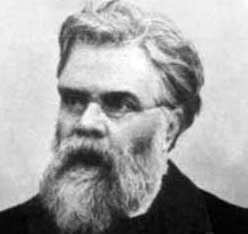The mathematician who first thought of this idea: Emile Lemoine
Lemoine's conjecture, sometimes referred to as Levy's conjecture, states that all odd numbers can be broken into the sum of an odd prime and an even semi-prime.

Here are three examples of "lemoine partitions", i.e. where the odd numbers are broken down into their constituent elements based on the definition above.
$$7 = 3 + 2 * 2$$
$$9 = 3 + 2 * 3$$
$$11 = 5 + 2 * 3$$
In 1999, Dann Corbit was able to verify the conjecture to 1,000,000,000 numbers utilizing the following code in C (https://groups.google.com/forum/?hl=en#!msg/sci.math/HoCpH8gPDHM/gtU-fuSWnFMJ):

#include <stdio.h>
#include <stdlib.h>
#include <string.h>
#include <time.h>
#define TEST( f, x ) ( *( f+( x )/16 )&( 1<<( ( ( x )%16L )/2 ) ) )
#define SET( f, x ) *( f+( x )/16 )|=1<<( ( ( x )%16L )/2 )

unsigned        ip(unsigned long j, unsigned char *d)
{
if (j == 1)
return 0;
if ((j % 2) == 0) {
if (j == 2)
return 1;
else
return 0;
} else
return (!(*(d + (j) / 16) & (1 << (((j) % 16L) / 2))) && j != 1);
}

int             main(int argc, char *argv[])
{
unsigned char  *feld = NULL,
*zzz = NULL;
unsigned long   teste = 1,
max,
mom,
hits = 1,
alloc;
time_t          begin;
unsigned char   quiet = 0;
if (argc > 1) {
max = atol(argv) + 10000;
if (argc > 2)
quiet = 1;
} else
max = 1000000000L;

zzz = feld = malloc(alloc = (((max -= 10000L) >> 4) + 1L));
if (feld) {
char            found;
memset(zzz, 0, alloc);
printf("Searching prime numbers to : %lu\n", max);
begin = time(NULL);
while ((teste += 2) < max)
if (!TEST(feld, teste)) {
++hits;
for (mom = 3L * teste; mom < max; mom += teste << 1)
SET(feld, mom);
}
printf(" %ld prime numbers found in %.2f secs.\n\n",
hits, difftime(time(NULL), begin));
{
long            o,
p,
q,
j;
for (o = 7; o <= max; o += 2) {
found = 0;
for (j = 2; j < o;) {
p = j;
q = o - 2 * p;
if (ip(p, feld) && ip(q, feld)) {
if (!quiet)
printf("%lu = 2*%lu + %lu\n", o, p, q);
found = 1;
break;
}
if (j == 2)
j++;
else
j += 2;
}
if (!found)
printf("*** Problem!! %lu\n", o);
}

free(feld);
printf(" Finished calculations in %.2f secs.\n\n",
difftime(time(NULL), begin));
}
} else {
puts("Memory allocation failure.");
exit(EXIT_FAILURE);
}
return 0;
}


A new verifier was implemented in Python to try and either match/exceed this number.

"""
Description: Traditional Implementation of Lemoine's Conjecture
"""

import numpy as np
import time

def primesfrom2to(n):
# https://stackoverflow.com/questions/2068372/fastest-way-to-list-all-primes-below-n-in-python/3035188#3035188
""" Input n>=6, Returns a array of primes, 2 <= p < n """
sieve = np.ones(n/3 + (n%6==2), dtype=np.bool)
sieve = False
for i in xrange(int(n**0.5)/3+1):
if sieve[i]:
k=3*i+1|1
sieve[      ((k*k)/3)      ::2*k] = False
sieve[(k*k+4*k-2*k*(i&1))/3::2*k] = False
return np.r_[2,3,((3*np.nonzero(sieve)+1)|1)]

def main():
start_time = time.time()
n = 7
x = 0
l = list(primesfrom2to(1000000000))
sl = set(l)
print("Done")
print("--- %s seconds ---" % (time.time() - start_time))

while (n < 1000000000):
if(n-(2*l[x]) in sl):
n = n+2
x = 0
x = x + 1
print("Done 2")
print("--- %s seconds ---" % (time.time() - start_time))

main()


This version relied on a pre-generated list of prime numbers in both list and set form to generate odd numbers sequentially. It rearranges the formal definition of Odd # = 2q + p to Odd # - 2q = p to take advantage of python's native set data type and enhanced searching capabilities. Replacing these with just lists, numpy arrays, or iterators was considered but not successfully implemented in a time-conserving manner.

You can track the progress of the generator by inserting this code snippet within the if statement within the while loop within the main method.

if n % 1000 == 1:
print("\r{}/{}".format(n, 100000))


Here is a table with the speed results from this implementation. The program reached 2*10^9 before stressing the hardware due to lack of available memory.

 Odd #’s up to ___ checked Time 10,000 --- 0.0129 seconds --- 100,000 --- 0.129 seconds --- 1,000,000 --- 1 seconds --- 10,000,000 --- 11 seconds --- 100,000,000 --- 138 seconds --- 1,000,000,000 --- 1593 seconds --- 2,000,000,000 --- 3,487 seconds ---

Another approach would be to generate the results from all of the combinations of p + 2q and match those up to a list of the odd numbers greater than or equal to seven. This was implemented with the following program.

"""
Description: New strategy to tackle verifying Lemoine's conjecture
"""

import numpy as np
import time

def primesfrom2to(n):
# https://stackoverflow.com/questions/2068372/fastest-way-to-list-all-primes-below-n-in-python/3035188#3035188
""" Input n>=6, Returns a array of primes, 2 <= p < n """
sieve = np.ones(n/3 + (n%6==2), dtype=np.bool)
sieve = False
for i in xrange(int(n**0.5)/3+1):
if sieve[i]:
k=3*i+1|1
sieve[      ((k*k)/3)      ::2*k] = False
sieve[(k*k+4*k-2*k*(i&1))/3::2*k] = False
return np.r_[2,3,((3*np.nonzero(sieve)+1)|1)]

arr1_e = np.repeat(np.expand_dims(arr1, 1), arr2.size, axis=1)
arr2_e = np.repeat(np.expand_dims(arr2,  0), arr1.size, axis=0)
return arr1_e + arr2_e

def main():
start_time = time.time()
list1 = primesfrom2to(1000000)
list2 = 2 * list1
list4 = np.arange(7,3000000,2)
print(list4)
print("--- %s seconds ---" % (time.time() - start_time))

main()


While the original versions of this implementation utilized nested for loops (later changed to for loop containing a map(add,primes,semiprimes) function with rolling numpy primes loop), Ernie Parke contributed the cartesian_add method which shaved off several seconds (it runs about 4x faster without the use of the np.unique function than the original implementation).

The cartesian_add function essentially generates all of the combinations of addition while the set.diff1d function figures out which numbers are only a part of the set of odd numbers (originally represented as list4) and therefore haven't been verified.Schematic of the principle utilized in the "new strategy." Source
Nevertheless, this version was able to only check 100,000 numbers in approx. five seconds which was much slower when compared to the original implementation (see the table above).

Jacob G. of Futuresight Technologies also developed a C++ code to to verify Lemoine's conjecture for a certain number of digits. Please note that it requires -fopenmp flag if you want to utilize the parallelism within the program to decrease run-time.

#include <iostream>
#include <vector>
#include <set>

typedef unsigned long long ull;

using namespace std;

const ull maxNumber = 1000000000;

bool numberWorks(ull n, vector<ull>& primes, set<ull>& primeSet) {
ull factor;
ull remainder;
ull primeSize = primes.size();
ull semiPrime;
for (ull i = 0; i < primeSize; i++) {
factor = primes[i];
if (factor > n) {
return false;
}
remainder = n - factor;

if (remainder % 2 == 0 && primeSet.find(remainder / 2) != primeSet.end()) {
return true;
}
}
return false;
}

int main() {
bool* primes = new bool[maxNumber];
bool* semiPrimes = new bool[maxNumber];

vector<ull> primeList;
set<ull> primeSet;

for (int i = 0; i < maxNumber; i++) {
primes[i] = true;
}

for (int i = 2; i < maxNumber; i++) {
if (primes[i]) {
for (int j = i; j < maxNumber; j += i) {
primes[j] = false;
}
primeList.push_back(i);
primeSet.insert(i);
}
}
cout << "Computed primes!" << endl;

for (ull numberToCheck = 7; numberToCheck < maxNumber; numberToCheck += 2) {
if (!numberWorks(numberToCheck, primeList, primeSet)) {
cout << "Number failed: " << numberToCheck << endl;
} else if ((numberToCheck - 1) % 1000000 == 0) {
cout << (numberToCheck / (double)maxNumber) * 100 << "%" << endl;
}
}

delete[] primes;
delete[] semiPrimes;
}


He contributed the following speed readings:

 Odd #’s up to ___ checked Time 1,000,000 --- .6 seconds --- 10,000,000 --- 15 seconds --- 100,000,000 --- 301 seconds ---

Out of curiosity, the original C code was run again to see how efficient it was. Due to C being a compiled language, this test ended up being more successful than the python programs at and allowed the conjecture to be verified to 10^10 in a reasonable time period. Here are some times for those runs:

 Odd #’s up to ___ checked Time 1,000,000,000 --- 108 seconds --- 10,000,000,000 --- 1009 seconds ---

Therefore, it is concluded that the conjecture is now verified up to 10^10 through the original C code, and up to 2*10^9 through the new Python implementation.

Thank you to Ernie Parke and Jacob G. answering all of my questions throughout this process.

Sources:
https://en.wikipedia.org/wiki/Lemoine%27s_conjecture
http://mathworld.wolfram.com/LevysConjecture.html
https://planetmath.org/levysconjecture
https://primes.utm.edu/curios/page.php?number_id=82&submitter=Capelle
https://www.mathsteacher.com.au/year7/ch11_ratios/04_prob/unit.htm
https://oeis.org/A046927

### The Lewis Dot Structure for HCNCreated by MakeTheBrainHappy

The molecule HCN has one of the most fascinating lewis structures. It is one of the few which features a nitrogen triple bond. It is possible to draw the structure with two electrons in place of the lines to represent the covalent bonds, which would result in there being six shared electrons between the carbon and nitrogen. We know that hydrogen can only share one bond with the carbon as we looked at in a previous article. Hydrogen essentially only needs two valence electrons to fill its outer shell because it only has a 1s orbital. The carbon, based on its position in the periodic table, has four electrons to share which it does with the nitrogen. The total number of "shared" electrons equals eight, filling carbon's valence shell. The nitrogen's "shared electron" count is six, which means that it needs two more electrons to fill its shell which is accounted for by the lone pair. Furthermore, this brings the total number of electrons on nitrogen to five as the periodic table predicts.

HCN has a linear geometry but unlike nonpolar CO2 is polar due to the intrinsic electronegativity on nitrogen and the stronger force by Coulomb's law pulling on the electrons in the triple bond creating a more negative bent on the right (based on the diagram) than the left. This means that it undergoes dipole-dipole interactions not unlike water (h2o). Water's intermolecular bonds are considered to be stronger hydrogen bonds (H-F, H-N, H-O bonds) due to these having the largest electronegativity difference. By this logic, HCN dipole-dipole interactions are also weaker the intramolecular bonds in network covalent solids. Nevertheless, these stronger intermolecular forces contribute to its boiling point at room temperature, or approximately 26˚C.Structure of HCN from Wikimedia Commons. Source

While HCN is produced as a precursor to many molecules and chemicals, the compound itself is extremely flammable and poisonous. It can be produced by combining hydrogen chloride (HCl) and Sodium Cyanide (NaCN). Often described as a pale or blue colorless liquid, it has a distinctive scent that will warn those of its toxicity. Furthermore, it is a weak acid that partially ionizes in water.

HCN's structure also features an sp hybridized structure with 180˚ of difference between the two molecules on the end. The triple bond contains one sigma bond and two pi bonds. Such a simple molecule can in the end still contain more complex chemistry.

Source:

### Primitive Data Types in Java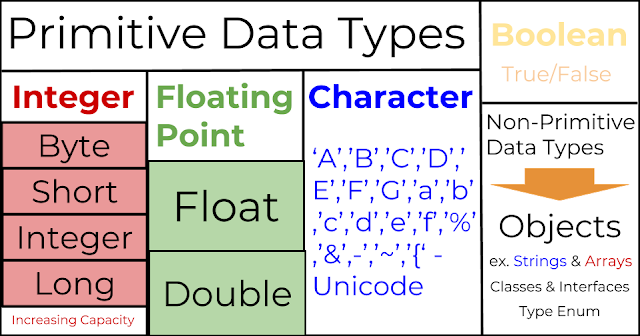Graphic with the eight primitive data types by MakeTheBrainHappy.
Data in computer science, especially in Java, it defined with a type that defines the type of data that can be stored (words, numbers, etc.) and the range of values (i.e. length of the words or size of numbers, etc.) that can be acted upon.

Primitives are built-in data types; other types are "reference" types that need to be defined in a class blueprint and "created" as objects. Primitives are simpler and will allow us to begin our discussion of data. Java has eight primitive data types (the three most common are starred):

Byte: integer value (8 bits)

Short: integer value (16 bits)

*Int: integer value (32 bits)

Long: integer value (64 bits)

Float: floating point # (32 bits)

*Double: floating point # (64 bits)

Character: one unicode character

*Booleans: a true or false value

A larger number of bits corresponds to a larger number which can be stored into those variables.

Declaring Primitives:

byte varName;
short VarName;
int varname;
long Varname;
float vArname;
double vArName;
char varNAme;
boolean varNAMe;


These variables can be then be assigned using the = operator to values fitting the descriptions for each of the primitive data types. If you add the keyword final between the type and variable name, then you have declared a constant (you cannot change the data within the variable later in the program).

Reserved Keywords:

Java has certain reserved keywords that cannot be utilized as variable names due to their special functions within the language. These are abstract, assert, boolean, break, byte, case, catch, char, class, const, default, do, double, else, enum, extends, false, final, finally, float, for, goto, if, implements, import, instanceof, int, interface, long, native, new, null, package,  private, protected, public, return, short, static, strictfp, super, switch, synchronized,  this, throw, throws, transient, true, try, void, volatile, while, and continue.

Visibility Modifiers:

Variables can either be declared as public or private, reserved keywords which are placed in front of the type. Public variables can be accessed by all classes while private variables can only be utilized by the class in which they are declared.

Static vs. Non-Static Variables:

This keyword, also placed before the type but after the visibility modifier, determines whether the variable is a part of the object declared from the class (thereby only being accessible through the identifiers of an object) as opposed to static variables are a part of the class and therefore can be accessed from "static" contexts such as the main method or static methods.

Casting

Some variables can be changed from one type to another through casting. Between the six types dealing with numerical values, you can cast utilizing the casting operator placed before the variable name. For example, here is a variable being converted from a integer to a double.

int varname = 1;
double newDouble = (double)varname;


This is a widening conversion since a more restrictive data type is changed to a more free version; therefore, no data is lost. But if you are converting a double to an integer, you would lose the information encoded by the decimals. This means that this would be a narrowing conversation.